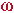Math & Science Home | Proficiency Tests | Mathematical Thinking in Physics | Aeronauts 2000

 CONTENTS Introduction Fermi's Piano Tuner Problem How Old is Old? If the Terrestrial Poles were to Melt... Sunlight Exerts Pressure Falling Eastward What if an Asteroid Hit the Earth Using a Jeep to Estimate the Energy in Gasoline How do Police Radars really work? How "Fast" is the Speed of Light? How Long is a Light Year? How Big is a Trillion? "Seeing" the Earth, Moon, and Sun to Scale Of Stars and Drops of Water If I Were to Build a Model of the Cosmos... A Number Trick Designing a High Altitude Balloon Pressure in the Vicinity of a Lunar Astronaut Space Suit due to Outgassing of Coolant Water Calendar Calculations Telling Time by the Stars - Sidereal Time Fields, an Heuristic Approach The Irrationality ofThe Irrationality ofThe Number (i)i Estimating the Temperature of a Flat Plate in Low Earth Orbit Proving that (p)1/n is Irrational when p is a Prime and n>1 The Transcendentality ofIdeal Gases under Constant Volume, Constant Pressure, Constant Temperature and Adiabatic Conditions Maxwell's Equations: The Vector and Scalar Potentials A Possible Scalar Term Describing Energy Density in the Gravitational Field A Proposed Relativistic, Thermodynamic Four-Vector Motivational Argument for the Expression-eix=cosx+isinx Another Motivational Argument for the Expression-eix=cosx+isinx Calculating the Energy from Sunlight over a 12 hour period Calculating the Energy from Sunlight over actual full day Perfect Numbers-A Case Study Gravitation Inside a Uniform Hollow Sphere Further note on Gravitation Inside a Uniform Hollow Sphere Pythagorean Triples Black Holes and Point Set Topology Additional Notes on Black Holes and Point Set Topology Field Equations and Equations of Motion (General Relativity) The observer in modern physics A Note on the Centrifugal and Coriolis Accelerations as Pseudo Accelerations - PDF File On Expansion of the Universe - PDF File

## Falling Eastward

Problem:
Let a test object be at an initial height h, small enough that the variation in the value of g (= 9.807 m/sec2) may be ignored. Calculate the eastward deflection due to the Coriolis effect if the object is allowed to free-fall from an initial state of rest to the earth's surface.

Solution:
Let= earth's angular velocity due to rotation. Then,(cos l) is the component of the angular velocity tangent to the earth's surface at latitude l, and is the component of concern to this problem since it is perpendicular to the plane of the test object's motion6. The Coriolis acceleration due to this component of angular velocity is

 2 v(cos l) 1

and is directed eastward. The term v is the velocity of the falling test object as a function of time. If the eastward deflection is assumed sufficiently small compared to h, we may approximate v and h as

 v = gt 2
and
 h = (1/2) gt2 3

Substituting 2. into 1. gives for the Coriolis acceleration

 2 gt(cos l) 4

Integrating once give the eastward velocity as a function of time

 vE = gt2(cos l) 5

Integrating a second time gives the required deflection

 xE = (1/3) gt3(cos l) 6

Using 3., t3 = (2h/g)3/2, so that for the entire fall from height h,

 xE = (2h)3/2 g-1/2(cos l) 7

Let us now assume that the initial height h = 100 m, and l = 42 deg. With= 7.27 x 10-7 /sec, we find that at the moment of impact,

t = 4.52 sec
v = 44.3 m/sec
vE = 1.08 x 10-4 m/sec
xE = 1.63 x 10-4 m = .16 mm

Comparing vE and v, we also see that the deviation of the velocity from the vertical is

arctan (1.08 x 10-4/44.3) = 1.4 x 10-4 deg.

With h = 1000 m, l = 42 deg, we find

t = 14.3 sec
v = 140 m/sec
vE = 1.08 x 10-3 m/sec
xE = 5.16 x 10-3 m = 5.16 mm
with arctan (1.08 x 10-3/140) = 4.4 x 10-4 deg.

6. The deviation of the test object's velocity vector from the vertical is assumed small enough to be ignored; hence, its cross product with the vertical component of the earth's angular velocity is also small enough to be ignored.